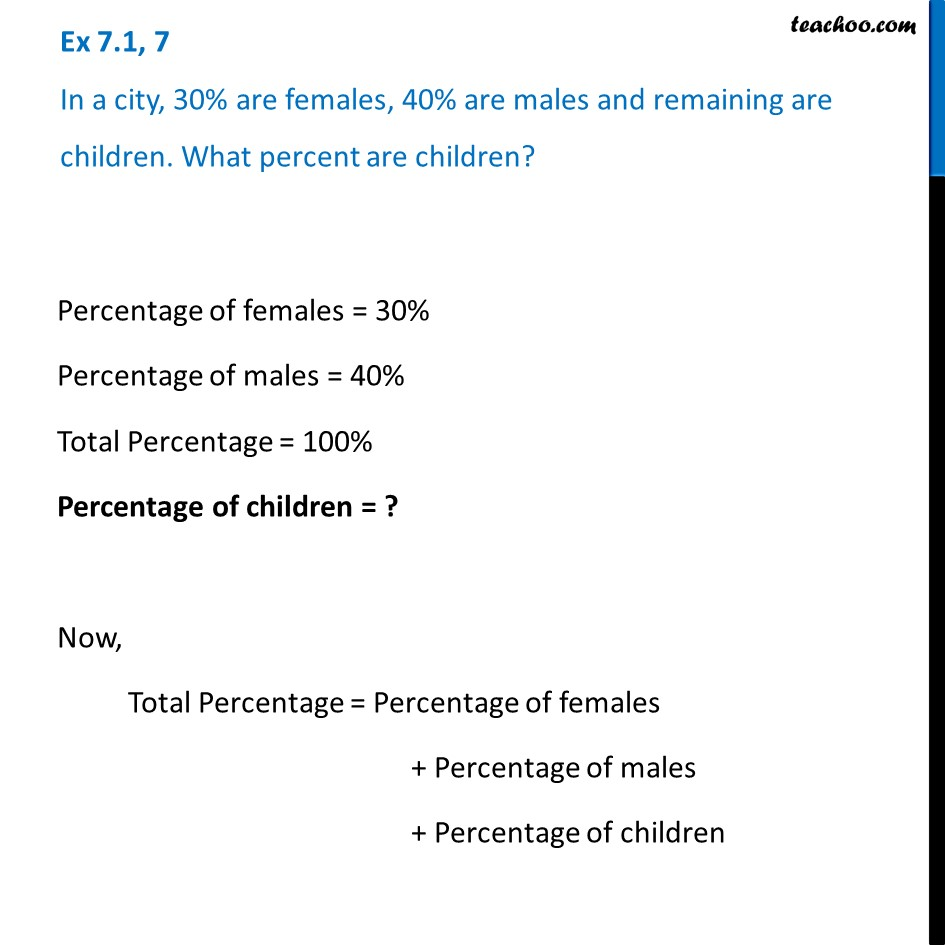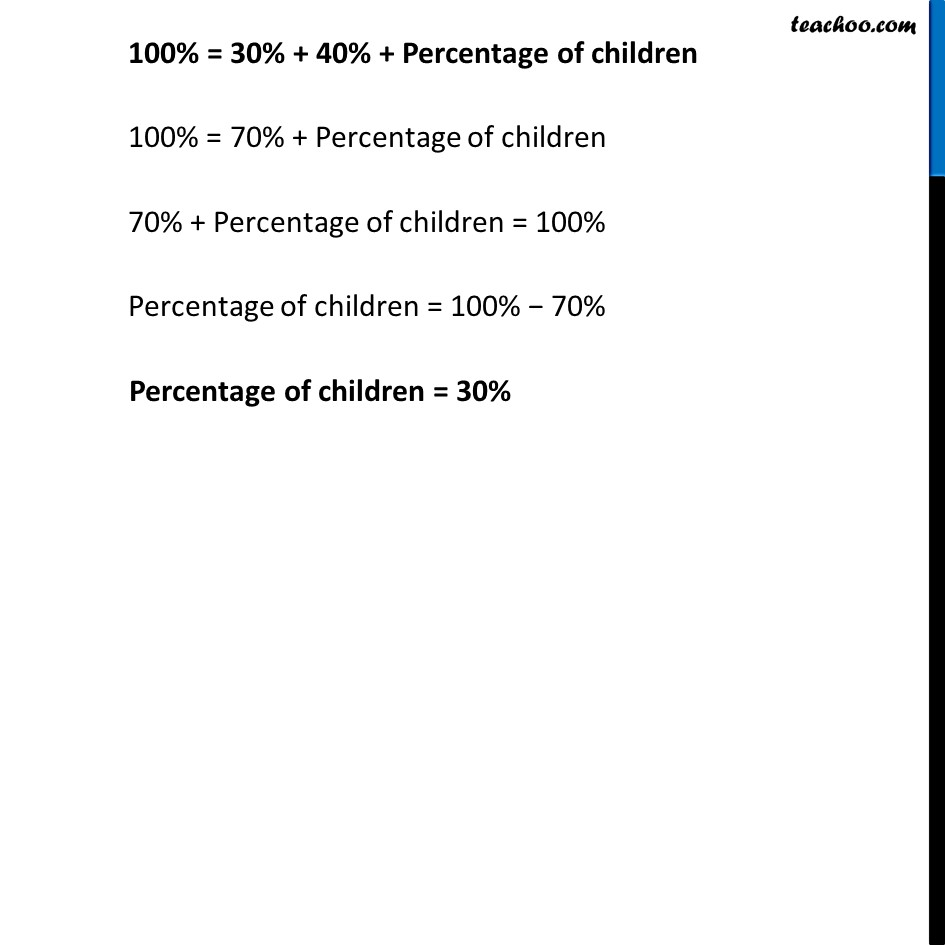Ex 7.1

Chapter 7 Class 7 Comparing Quantities
Serial order wiseLearn in your speed, with individual attention - Teachoo Maths 1-on-1 Class

### Transcript

Ex 7.1, 7 In a city, 30% are females, 40% are males and remaining are children. What percent are children? Percentage of females = 30% Percentage of males = 40% Total Percentage = 100% Percentage of children = ? Now, Total Percentage = Percentage of females + Percentage of males + Percentage of children 100% = 30% + 40% + Percentage of children 100% = 70% + Percentage of children 70% + Percentage of children = 100% Percentage of children = 100% − 70% Percentage of children = 30%